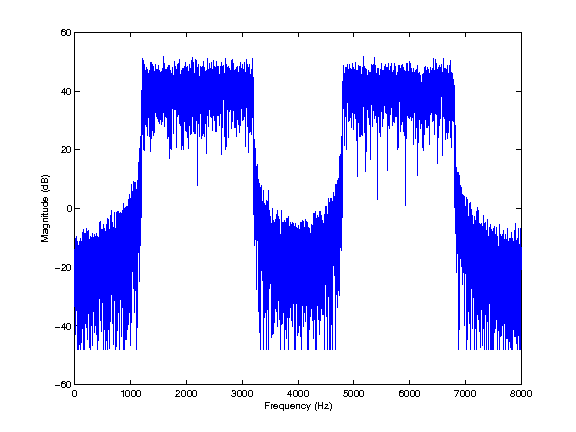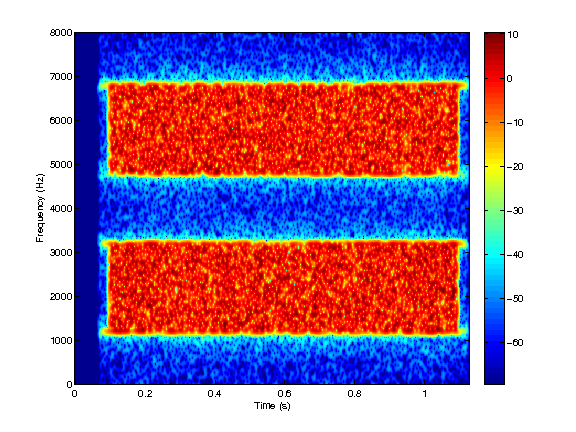# THE AUDITORY MODELING TOOLBOX

Applies to version: 0.9.7

Go to function

# NOTCHEDNOISE - Generates a notched-noise-type masker

## Usage

outsig = notchednoise(fs,fc,dur,L,bw,delta);
outsig = notchednoise(fs,fc,dur,L,bw,[deltaL deltaR]);


## Description

outsig = notchednoise(fs,fc,dur,L,bw,delta) generates a notched-noise masker with duration dur (in sec) and overall level L (in dB SPL) with a sampling rate of fs Hz. The deviation from center frequency fc is symmetric and is given by delta such that the stopband is [fc-delta*fc fc+delta*fc]. The left and right noise bands have a bandwidth of bw*fc in Hz. If delta=0 then a broadband noise is returned.

outsig = notchednoise(fs,fc,dur,L,bw,[deltaL deltaR]) generates a notched-noise masker with an asymmetric configuration. deltaL and deltaR denote the left and right deviations from fc, respectively. In this case the stopband is [fc-deltaL*fc fc+deltaR*fc].

This notched-noise-type masker was used in psychoacoustical studies investigating the auditory filters' shape (the original method is described in Patterson, 1974). The noise is composed of two noise bands of width bw and a stopband centered at fc with a deviation from fc given by delta.

## Examples:

The following shows the spectrum and a spectogram of a typical notched noise masker used in the Patterson study:

fc    =  4000;
fs    = 16000;
dur   =     1;
L     =   100;
bw    =    .5;
delta =    .2;
outsig = notchednoise(fc,fs,dur,L,bw,delta);

figure(1);
plotfftreal(fftreal(outsig),fs,100);

figure(2);
sgram(outsig,fs,80);## References:

B. Moore, R. Peters, and B. Glasberg. Auditory filter shapes at low center frequencies. J. Acoust. Soc. Am., 88(1):132-140, 1990.

R. Patterson. Auditory filter shape. J. Acoust. Soc. Am., 55:802-809, 1974.